# Normal Distribution in Excel: Finding Area

Joshua earned an MBA from USF and he writes mostly about software and technology.

## Normal Distribution

The normal distribution is a very common continuous probability distribution in probability theory. The normal distribution is often referred to as the bell curve, even though other distributions have a bell-shaped curve as well. This distribution is useful because properties of the central limit theorem allow for its use in many applications.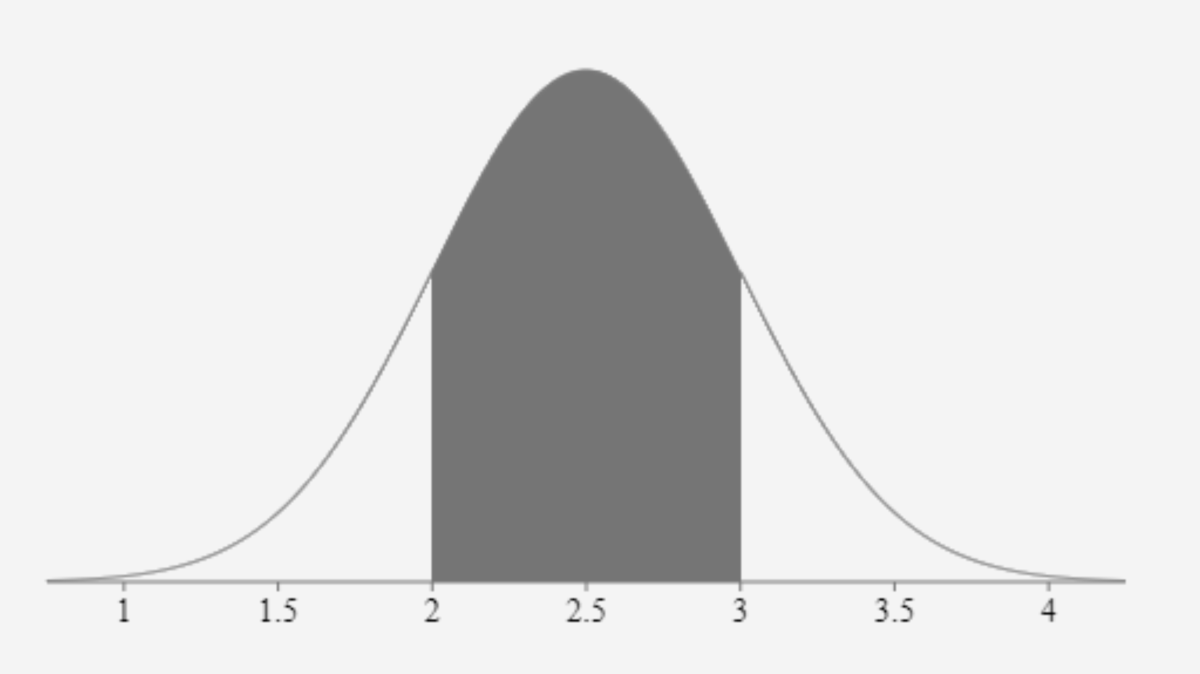A normal distribution is also known as Gaussian, Gauss, or the Laplace–Gauss distribution. Created by Joshua Crowder

## Excel Normal Distribution Function (NORM.DIST Function)

The normal distribution function displays the normal distribution when a specified mean, standard deviation, x value, and function determination are added to the function. The use of this function is found mostly in statistics to determine the area in a normal distribution. Hypothesis testing, which is in the inferential area of statistics, is where you will find wide use of this function. A description of the NORM.DIST syntax is displayed in the table below.

## Syntax for the Normal Distribution Function

Take note that for this formula to work an equals sign needs to be added before the function name. Also, the variables of the function need to be separated by a comma and located within parenthesis.

=NORM.DIST(x,mean,standard_dev,cumulative)

x = The value to be tested

mean = The arithmetic mean of the distribution

standard_dev = The standard deviation of the distribution

cumulative = FALSE or zero, is the prob. that x will occur

cumulative = TRUE or non-zero, is the prob. of less than or equal to x will occur

## Left Tail Example

Let's just say for example that a company has an average sales value of 2.5 million dollars. Also, let’s suppose that sales are normally distributed with a standard deviation of .5 million and we want to know the percentage of sales below 3.5 million. When we plug our numbers into a cell =NORM.DIST(3.5,2.5,.5,true) we get .977 as a result. True is selected for the type of function because we want to see the cumulative distribution up to the point of 3.5.

Since the example states that we want to find the percentage of sales below or less than 3.5, we know we are calculating the percentage in the left tail. When this function is used to calculate the left tail no further calculations are permitted after the NORM.DIST function is used.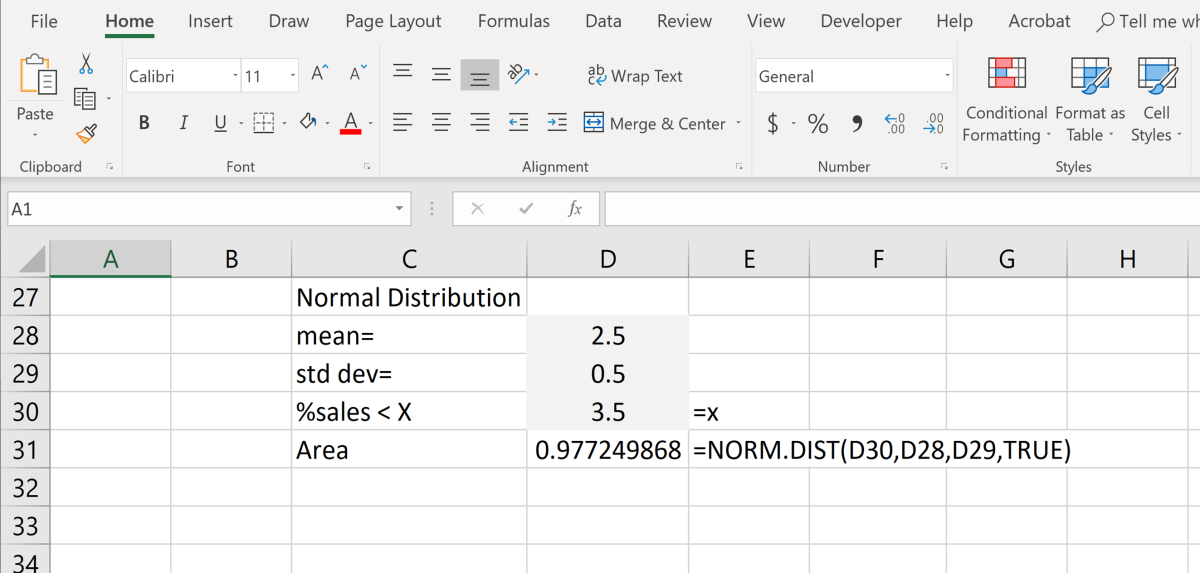Since we are looking for what is less than x in the example, we are looking for the area to the left of our x value.Created by Joshua Crowder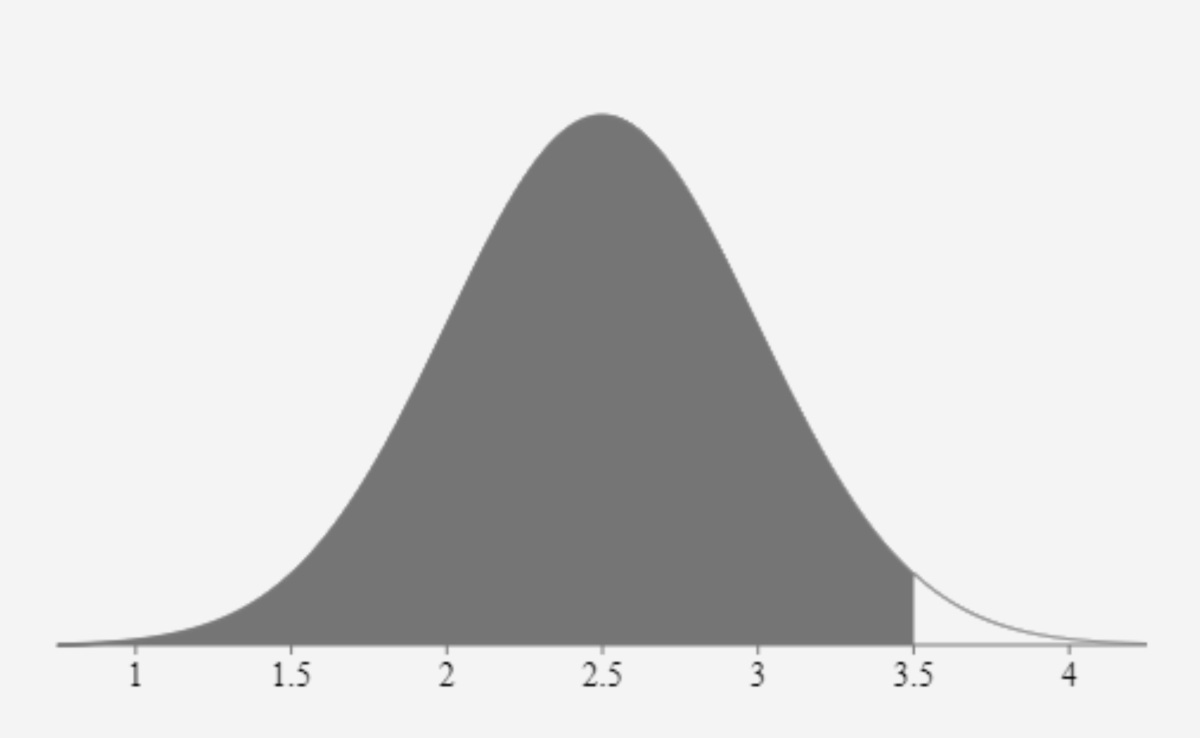The shaded area in this distribution shows the area of .977 that was calculated. The area to the right can now be calculated with this operation 1 - .977 = .023Created by Joshua Crowder

## Right Tail Example

This example is similar to the left tail example because the same data is being used. The average sales value is 2.5 million dollars, sales are normally distributed, the standard deviation is .5 million dollars and the value of x is 3.5 million dollars. The difference here is that we want to find the percentage of sales above 3.5 million.

Scroll to Continue

Essentially, to be able to find this value, we must find the same cumulative distribution as the previous example and subtract it from 1. So, the function appears like this: =1-NORM.DIST(3.5,2.5,.5,true). Since we are looking for everything in the right tail (greater than 3.5), all that needs to be calculated is the complement of the left tail.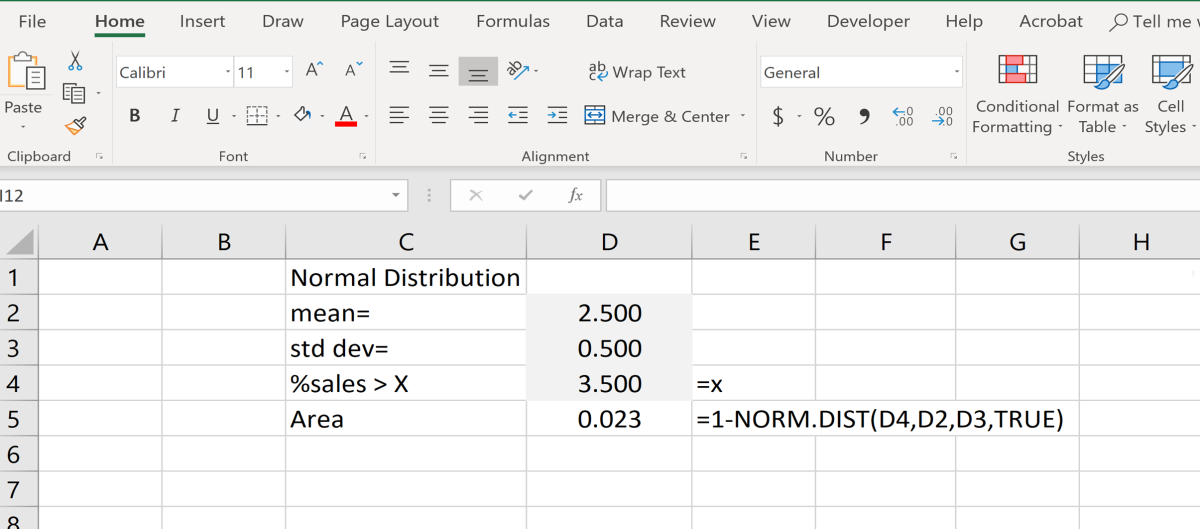Notice that the example above shows cell references in the function instead of the actual numbers. As you are creating your excel function you can click in the cell of the value you want instead of typing the actual value to build the function fasterCreated by Joshua Crowder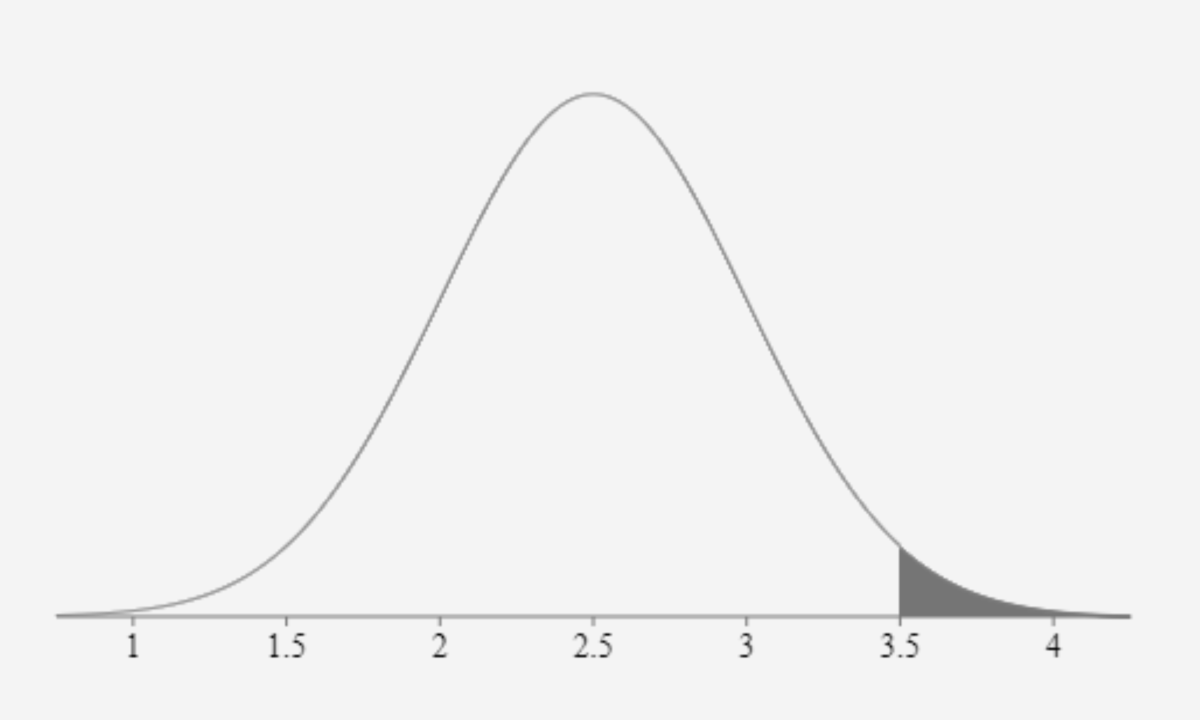To calculate the right tail, you must first calculate the left tail and subtract that function from 1. The shaded area above shows the area (.023) that was calculated for the right tail.Created by Joshua Crowder

## Center Area Example

The center area may seem tricky to calculate to some people. In this example, let's say we are still using 2.5 million dollars for average sales, sales are normally distributed, and the standard deviation is .5 million dollars. The only difference now is that we need to find the percentage of sales between 2 and 3.5 million dollars.

By looking at the previous distributions illustrations above can you figure out how to find the center area? You simply must find the area up to 3.5 million dollars, then subtract that area by the area that appears below 2. The operation of the function, in this case, looks like this =NORM.DIST(3.5,2.5,.5,true)-NORM.DIST(2,2.5,.5,true). This is self-explanatory. By subtracting the whole area to the left of 3.5 by the area to the left of 2 you will be left with the center.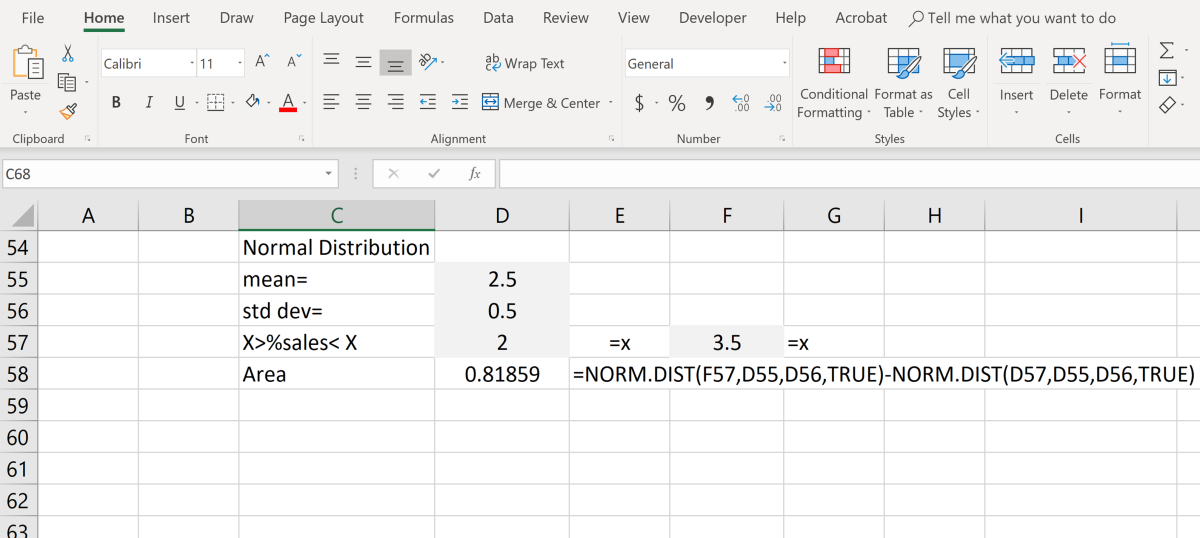To find the total area for each of the tails you could subtract the area in the center from one.Created by Joshua Crowder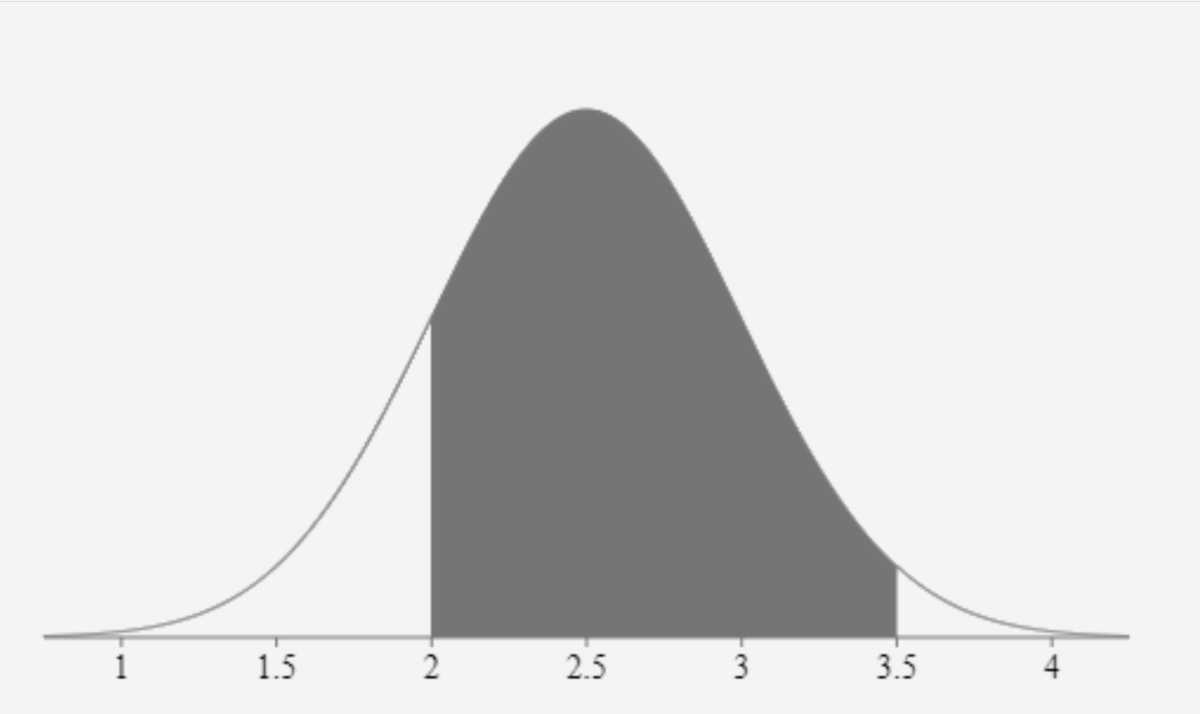The shaded in the normal distribution above is the result of subtracting the lower tail from the cumulative distribution of 3.5. Created by Joshua Crowder

To learn more about completing statistical calculations in Excel I suggest that you try reading the Excel Bible. This is a good reference book for beginner users of Excel.

• NORM.DIST function. (n.d.). Retrieved October 15, 2018, from https://support.office.com/en-us/article/norm-dist-function-edb1cc14-a21c-4e53-839d-8082074c9f8d
• Normal distribution. (2018, October 10). Retrieved October 15, 2018, from https://en.wikipedia.org/wiki/Normal_distribution

This content is accurate and true to the best of the author’s knowledge and is not meant to substitute for formal and individualized advice from a qualified professional.